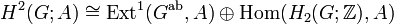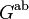# Second cohomology group for trivial group action of A4 on Z2

This article gives information about the second cohomology group for trivial group action (i.e., the second cohomology group with trivial action) of the group alternating group:A4 on cyclic group:Z2. The elements of this classify the group extensions with cyclic group:Z2 in the center and alternating group:A4 the corresponding quotient group. Specifically, these are precisely the central extensions with the given base group and acting group.
Get more specific information about alternating group:A4 |Get more specific information about cyclic group:Z2

## Description of the group$\! H^2(G;A)$

where$G$ is alternating group:A4 (i.e., the alternating group on a set of size four) and$A$ is cyclic group:Z2.

The cohomology group is isomorphic to cyclic group:Z2.

## Computation in terms of group cohomology$H^2(G;A) \cong \operatorname{Ext}^1(G^{\operatorname{ab}},A) \oplus \operatorname{Hom}(H_2(G;\mathbb{Z}),A)$

For$G$ the alternating group of degree four, we have, by group cohomology of alternating group:A4, that$G^{\operatorname{ab}}$ (the abelianization, also the first homology group) is cyclic group:Z3 and$H_2(G;\mathbb{Z})$ (the Schur multiplier) is cyclic group:Z2. Plugging in, we get:$H^2(G;A) \cong \operatorname{Ext}^1(\mathbb{Z}/3\mathbb{Z},\mathbb{Z}/2\mathbb{Z}) \oplus \operatorname{Hom}(\mathbb{Z}/2\mathbb{Z},\mathbb{Z}/2\mathbb{Z}) = \mathbb{Z}/2\mathbb{Z}$

## Elements

Cohomology class type Number of cohomology classes Corresponding group extension Second part of GAP ID (order is 24)
trivial 1 direct product of A4 and Z2 13
nontrivial 1 special linear group:SL(2,3) 3

## Cocycles and coboundaries

### Size information

We first give some quantitative size information if we use non-normalized cocycles and coboundaries:

Group Dimension as vector space over field:F2 Order of group (equals 2 to the power of dimension) Isomorphism class of group Explanation
group of 1-cocycles for trivial group action$Z^1(G;A)$ 0 1 Klein four-group Same as$\operatorname{Hom}(G,A)$. see first cohomology group for trivial group action is naturally isomorphic to group of homomorphisms
group of all 1-cochains for trivial group action$C^1(G;A)$ 12 4096 elementary abelian group of order 4096 all set maps from$G$ to$A$ with pointwise addition, so the dimension is the cardinality of$G$.
group of all 2-coboundaries for trivial group action$B^2(G;A)$ 12 4096 elementary abelian group of order 4096 By the first isomorphism theorem and the definition of this group, it is isomorphic to the group (1-cochains)/(1-cocycles), so the dimensions as vector spaces subtract and the orders divide.
group of all 2-cocycles for trivial group action$Z^2(G;A)$ 13 8192 elementary abelian group of order 8192
second cohomology group for trivial group action 1 2 cyclic group:Z2 This is$Z^2/B^2$, so dimensions subtract and orders divide.